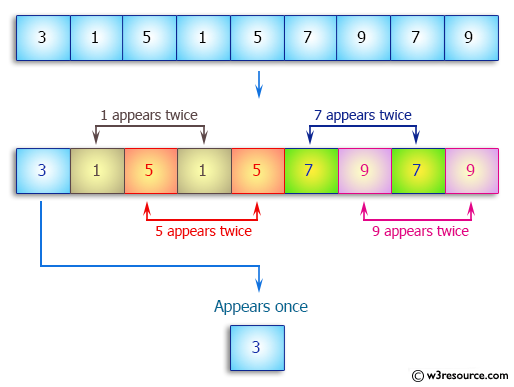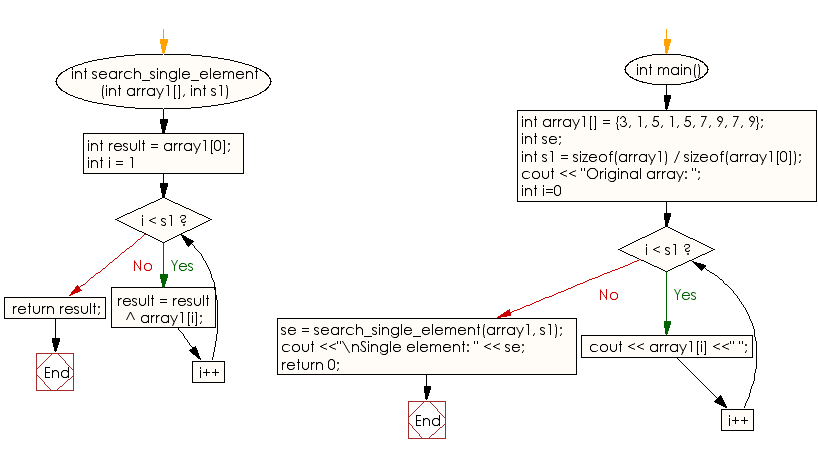﻿ C++ : Find an element appears once in an array, other twice# C++ Exercises: Find the element that appears once in an array of integers and every other element appears twice

## C++ Array: Exercise-23 with Solution

Write a C++ program to find the element that appears once in an array of integers and every other element appears twice.

Pictorial Presentation:Sample Solution:

C++ Code :

``````#include <iostream>
using namespace std;

int search_single_element(int array1[], int s1)
{
int result = array1;
for (int i = 1; i < s1; i++)
result = result ^ array1[i];

return result;
}

int main()
{
int array1[] = {3, 1, 5, 1, 5, 7, 9, 7, 9};
int se;

int s1 = sizeof(array1) / sizeof(array1);

cout << "Original array: ";

for (int i=0; i < s1; i++)
cout << array1[i] <<" ";

se = search_single_element(array1, s1);
cout <<"\nSingle element: " << se;
return 0;
}
``````

Sample Output:

```Original array: 3 1 5 1 5 7 9 7 9
Single element: 3
```

Flowchart:C++ Code Editor:

What is the difficulty level of this exercise?

Test your Programming skills with w3resource's quiz.

﻿